Question

# I want to know what is wrong with this code, and how to fix it please....

I want to know what is wrong with this code, and how to fix it please. i keep getting

Error using contour (line 48)
Z must be at least a 2x2 matrix.

Error in Projectrevised (line 42)
curve= contour(b,FOS1,h,'ShowText','on');

%% Constants
w = 450000;
l = 10;
n = 6;
SOM = 505000000;
max = 10;
min = 2;
diff = (max-min)/n;
FOS1 = zeros(n,n);
FOS2 = zeros(n,n);
i = 1;
j = 1;
Vm = (w*l)/2;

%% FOS@different base&height values

for b = min:diff:max
for h = min:diff:max
c = (h/2);
density=8000;
V=b*h*l;
mass=density*V;
Mmax = ((w*l^2)/8)*c;
I = (b*h^3)/12;
Bm = (Mmax*c)/I;
Tmax=(3*Vm)/2*(b*h);
Stressmax=(Mmax*c)/I;
FOS1(i,j) = (SOM/Tmax);
FOS2(i,j) = (SOM/Stressmax);
j = j+1;
end
i = i+1;
end

%% Plotting
[B,H]=meshgrid(b,h);
FOS1(i,j) = (SOM/Tmax);
curve= contour(b,FOS1,h,'ShowText','on');
hold on
title('Beam Design')
xlabel('Base (meters)')
ylabel('Height (meters)')
close all
clear plot

If you are plotting a contour plot and plotting z against x and y, then z needs to be a matrix and not a 1 D array.

You are using h to be plotted on z axis, which is an array. However, i see you have converted array h to a matrix H, using meshgrid. So instead of using h, use H.

The command should be

curve= contour(b,FOS1,H,'ShowText','on');

#### Earn Coins

Coins can be redeemed for fabulous gifts.

Similar Homework Help Questions
• ### Can you please help me with this question. Much appreciated. So I have a sample code,...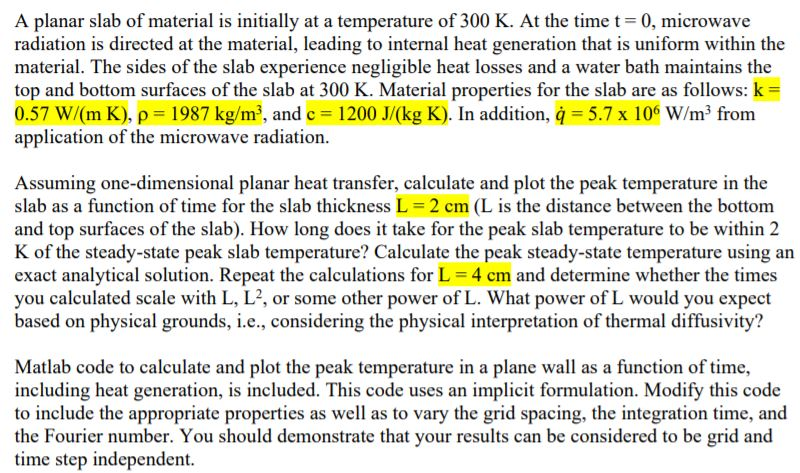Can you please help me with this question. Much appreciated. So I have a sample code, but we need to change the code in a way that fits the problem. A planar slab of material is initially at a temperature of 300 K. At the time t=0, microwave radiation is directed at the material, leading to internal heat generation that is uniform within the material. The sides of the slab experience negligible heat losses and a water bath maintains the...

• ### I keep getting this error and I do not know how to fix it. this is...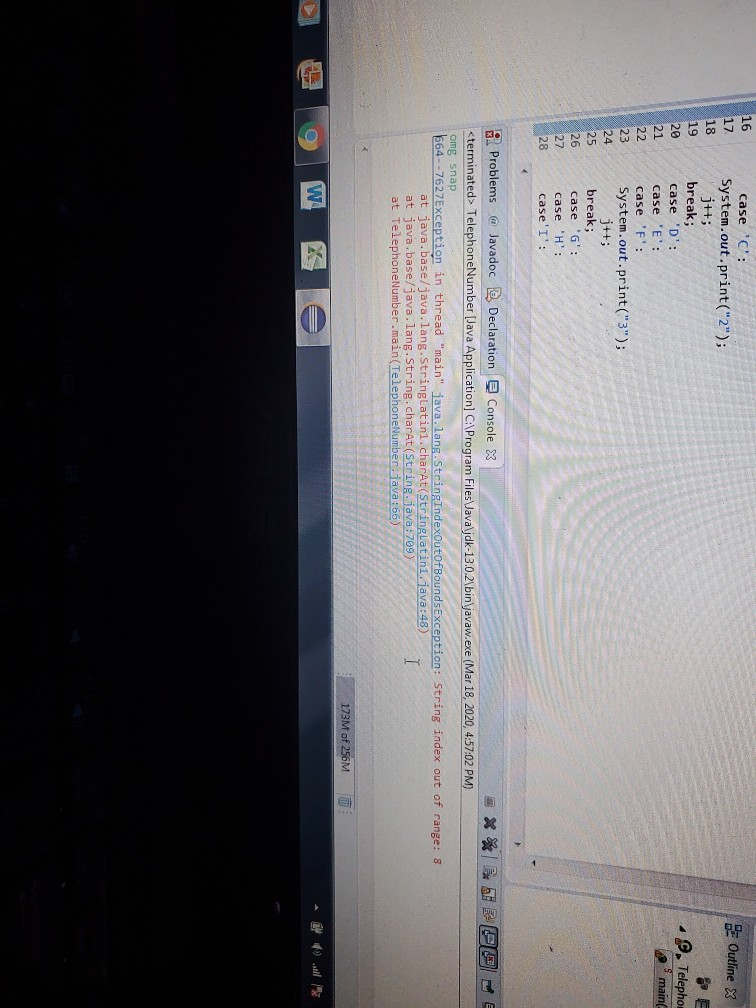I keep getting this error and I do not know how to fix it. this is the code I've been working on: please help me import java.util.*; public class TelephoneNumber { public static void main(String[] args) {String number; int i=0,j=0; char c; Scanner in=new Scanner (System.in); System.out.println("Enter the phone number: "); number=in.nextLine(); number=number.toUpperCase(); c=number.charAt(i); while(c!='\n'&&j<=7) {switch(c) {case 'A': case 'B': case 'C': System.out.print("2"); j++; break; case 'D': case 'E': case 'F': System.out.print("3"); j++; break; case 'G': case 'H': case'I': System.out.print("4");...

• ### I know that my work is correct. I know that delta U is not 0, and...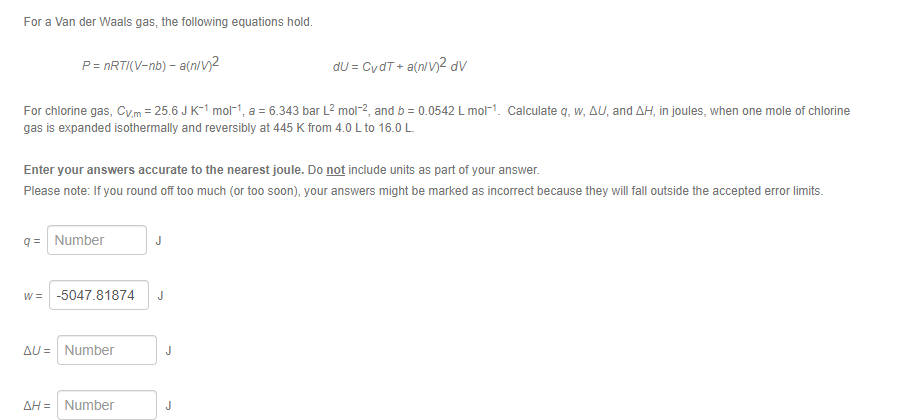I know that my work is correct. I know that delta U is not 0, and delta H is not 0. How do I complete this problem? For a Van der Waals gas, the following equations hold. P= nRTIV-nb) - a[n/v2 dU = CydT+ a(n/V)2 dv For chlorine gas, Cvm = 25.6 JK-1 mol-t, a = 6.343 bar L2 mol-2, and b = 0.0542 L molt Calculate q, w, AU, and AH, in joules, when one mole of chlorine gas...

• ### C++: Need help debugging my code I am writing this and using some other examples as reference code while rewriting it with my own understanding of the material but am having trouble making it finally...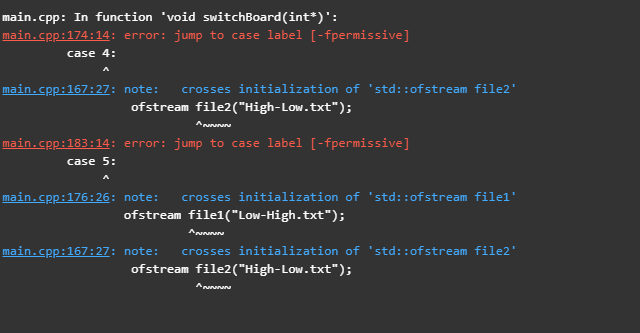C++: Need help debugging my code I am writing this and using some other examples as reference code while rewriting it with my own understanding of the material but am having trouble making it finally compile. Below is a picture of the error messages. //main.cpp //Semester Project //Created by J---on 5/6/2019 #include <iostream> #include <fstream> #include <string> #include <sstream> #include <bits/stdc++.h> using namespace std; void instructions(); //displays program details and instructions void openFile(); void takeInput(int*); void switchBoard(int*); struct price {...

• ### I have all of the answers to this can someone just actually explain this matlab code and the results to me so i can get a better understanding? b) (c) and (d) %% Matlab code %% clc; close all; clear...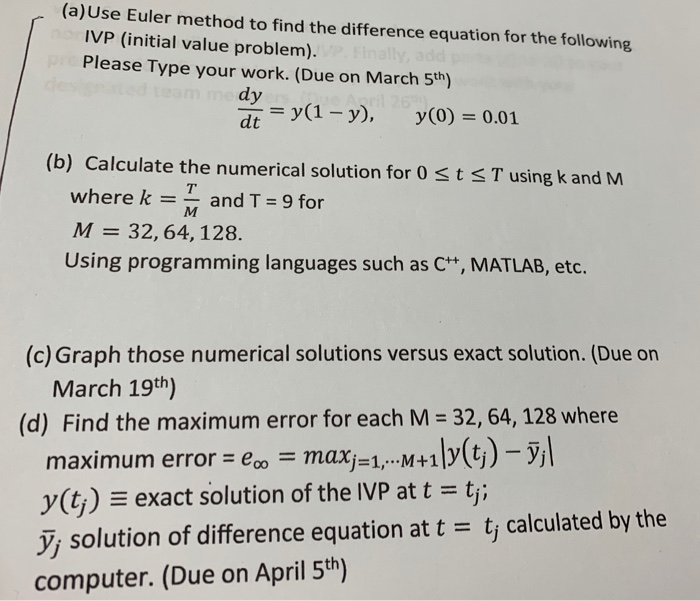I have all of the answers to this can someone just actually explain this matlab code and the results to me so i can get a better understanding? b) (c) and (d) %% Matlab code %% clc; close all; clear all; format long; f=@(t,y)y*(1-y); y(1)=0.01; %%%% Exact solution [t1 y1]=ode45(f,[0 9],y(1)); figure; plot(t1,y1,'*'); hold on % Eular therom M=[32 64 128]; T=9; fprintf(' M Max error \n' ); for n=1:length(M) k=T/M(n); t=0:k:T; for h=1:length(t)-1 y(h+1)=y(h)+k*f(t(h),y(h)); end plot(t,y); hold on %%%...

• ### the code in the photo for this I.V.P dy/dx= x+y. y(0)=1 i need the two in the photo thank you New folder Bookmark...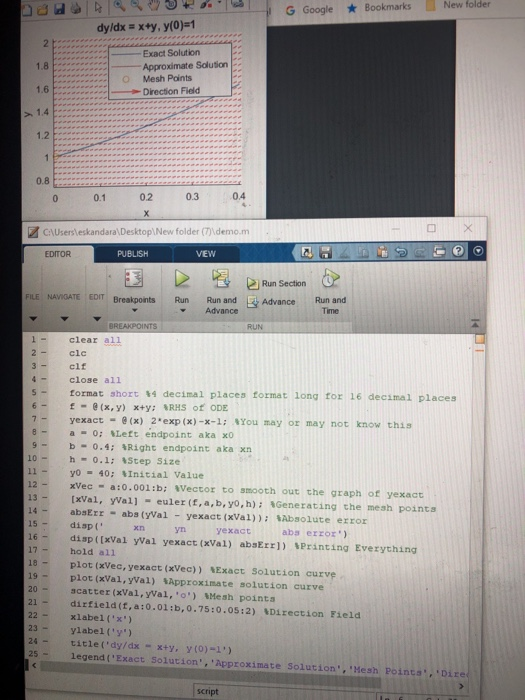the code in the photo for this I.V.P dy/dx= x+y. y(0)=1 i need the two in the photo thank you New folder Bookmarks G Google dy/dx x+y, y(0)=1 2 h Exact Solution 1.8 Approximate Solution Mesh Points 1.6 -Direction Fied 1.4 1.2 1 0.8 04 0.2 0.3 0.1 0 X CAUsersleskandara\Desktop\New folder emo.m EDITOR PUBLISH VEW Run Section FILE NAVIGATE EDIT Breakpoints Run Run and FL Advance Run and Advance Time BREAKPOINTS RUN 1 - clear all 2 clc 3-...

• ### I don't know how to terminate the code in the second time that whether or not...

I don't know how to terminate the code in the second time that whether or not the users want to continue to get a new number. PLEASE HELP. To make telephone numbers easier to remember, some companies use letters to show their telephone number. For example, using letters, the telephone number 438-5626 can be shown as GET LOAN. In some cases, to make a telephone number meaningful, companies might use more than seven letters. For example, 225-5466 can be displayed...

• ### Help me!! Please solve (1) and (2). And I desperately want to know how to solve (2) by using MATLAB. Please teach me Ma...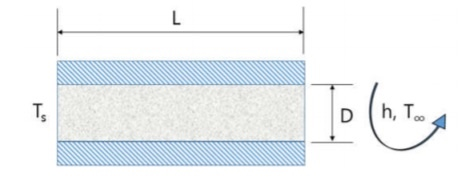Help me!! Please solve (1) and (2). And I desperately want to know how to solve (2) by using MATLAB. Please teach me Matlab code in detail. a rectangular shape Aluminum block (L=10mm, D=3mm) has well insulated top and bottom. The left surface has thermal boundary condition, and the right surface has convection boundary condition. * the surface temperature Ts=100℃, the ambient air temperature Ta=20℃, heat transfer coefficient h=120 W/(m^2*K) * thermal conductivity of Aluminum = 220 W/m*K, density of...

• ### how do I write this code without the imports? I don't know what pickle is or...

how do I write this code without the imports? I don't know what pickle is or os.path import pickle # to save and load history (as binary objects) import os.path #to check if file exists # character value mapping values = {'A': 1, 'B': 3, 'C': 3, 'D': 2, 'E': 1, 'F': 4, 'G': 2, 'H': 4, 'I': 1, 'J': 8, 'K': 5, 'L': 1, 'M': 3, 'N': 1, 'O': 1, 'P': 3, 'Q': 10, 'R': 1, ' S': 1,...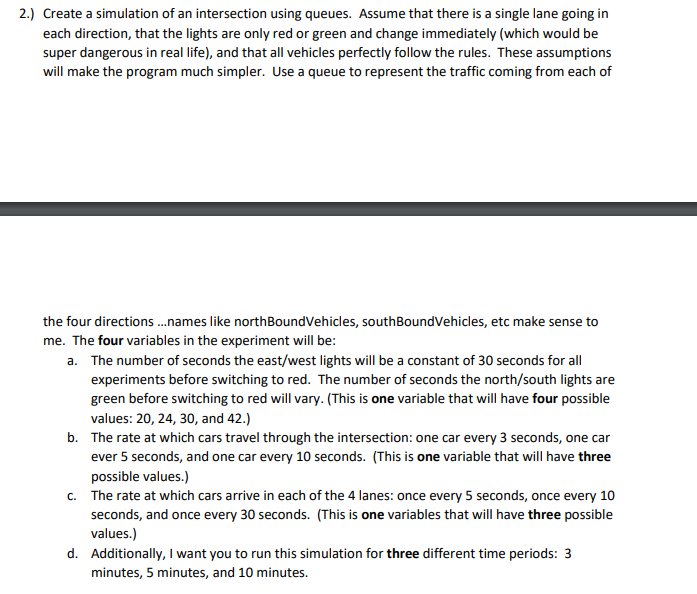Please help with my car traffic simulator! Code that I already have below, I do not know how to start it off! public class IntersectionSimulation { private final static int EAST_WEST_GREEN_TIME = 30 ; private final static int[] NORTH_SOUTH_GREEN_TIMES = { 20, 24, 30, 42 } ; private final static int[] CAR_INTERSECTION_RATES = { 3, 5, 10 } ; private final static int[] CAR_QUEUEING_RATES = { 5, 10, 30 } ; private final static int[] EXPERIMENT_DURATIONS = { 3*60, 5*60,...